Computer Science

# Practice with Bit

In your `cs110` project, you should have a folder called `bit`. Follow the guide on Introduction to Bit if you didn’t do this yet.

## First practice problem

Inside of your `bit` folder, create a new file called `practice.py`. Put this code in that file:

``````from byubit import Bit

@Bit.empty_world(5, 3)
def go(bit):
pass

if __name__ == '__main__':
go(Bit.new_bit)``````

This is starter code, meaning we have given you a piece of a program but you need to fill in the rest. This is an example of the types of problems we will give you when you write code using Bit. We will give you starter code and you need to fill in the rest.

In this problem, Bit starts in a blank 5x3 world: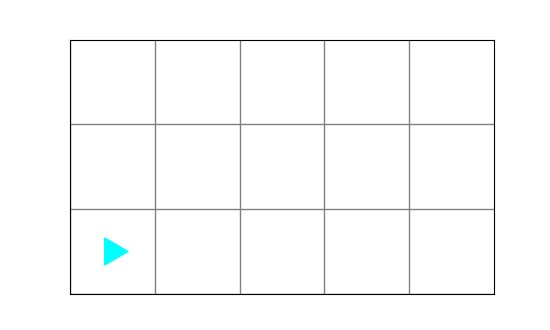Write code so that Bit finishes in a world like this:To do this, you only need to write code in the `go()` function definition.

Whenever you see a function that has one statement in it — `pass` — this is a function where you need to write code. The `pass` statement is a special keyword in Python that does literally nothing. It just exists as a placeholder for the code you need to write. Delete the `pass` keyword and write your code there.

In the guide, we will use this picture to indicate that you should pause and work out this problem a friend:Hopefully you wrote code with a friend! Since this is a guide, we will now give you the answer to this problem. It will be really tempting to just skip to the answers. Don’t do that!Remember, your whole purpose in life is to grow to be like Christ, and knowledge is a key part of being like Christ.

What if I don’t have a friend? Go to the lab! This is the whole purpose of lab sections, to give you an opportunity to make friends and learn to code together. If this is somehow not feasible for you, use the online lab sections in Discord. You can start your own video chat with a friend on Discord any time you want to code together.

OK, now here is the code for this problem:

``````@Bit.empty_world(5, 3)
def go(bit):
bit.move()
bit.move()
bit.move()
bit.left()
bit.move()
bit.paint("green")``````

We tell Bit to move forward three squares, turn left, move forward one square, and then paint green.

## Practice with errors #1

In your `bit` folder, create a new file called `no_cake.py` and put this code in it:

``````from byubit import Bit

@Bit.empty_world(5,3)
def make_a_cake(bit):
bit.move()
bit.paint('red')
bit.move()
bit.paint('green')``````
1. Draw out what you think this code should do.
2. Can you run this code? Why not?Hopefully you drew out something like this:Maybe you noticed that you can’t run this code because there is no main block. To run the code, you need to add this to the end:

``````if __name__ == '__main__':
make_a_cake(Bit.new_bit)``````

Once you add this, you will see the green triangle appear to the left, allowing you to run the code.

## Practice with errors #2

In your `bit` folder, create a new file called `get_moving.py` and put this code in it:

``````from byubit import Bit

@Bit.empty_world(5, 3)
def main(bit):
bit.move
bit.move
bit.paint("green")

if __name__ == '__main__':
main(Bit.new_bit)``````

If you run this code, what do you think will happen?Now run the code, and you should see this:Can you see why `bit.move` doesn’t do anything? The code is missing the `()`. Remember, `bit.move()` is a function, and you need to use parentheses whenever you call a function.

Try adding the parentheses and re-running the code.

## Practice with errors #3

In your `bit` folder, create a new file called `go_the_distance.py` and put this code in it:

``````from byubit import Bit

@Bit.empty_world(5, 3)
def go_go_go(bit):
bit.move()
bit.move()
bit.move()
bit.move()
bit.move()
bit.paint('green')

if __name__ == '__main__':
go_go_go(Bit.new_bit)``````

If you run this code, what do you think will happen?Now run the code and you should see this: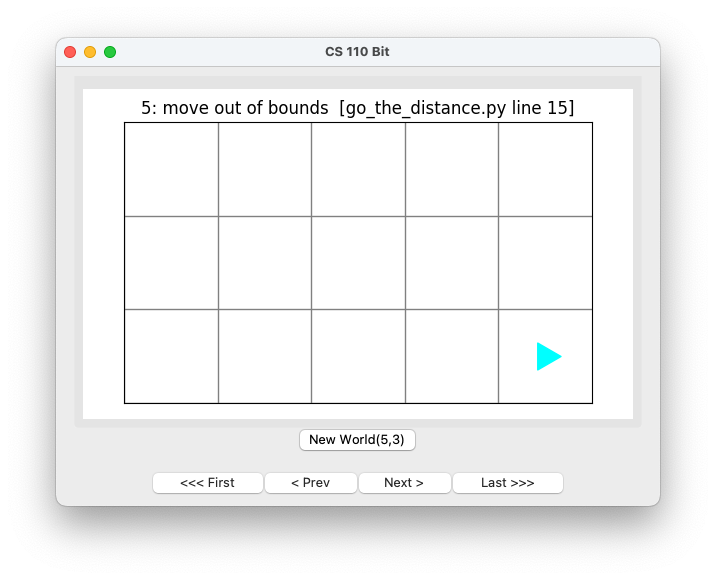The top of the window will show that you tried to move “out of bounds” meaning outside of the 5x3 Bit world. You will also see an error in the bottom part of the screen in PyCharm: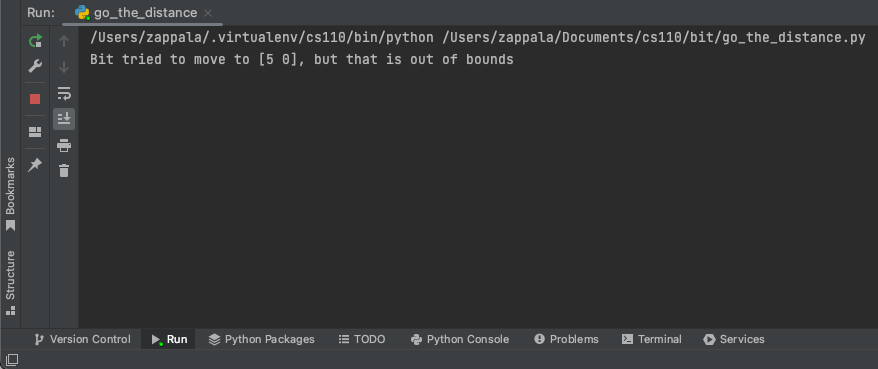Your program will stop running at the point that you ask Bit to move out of bounds, and nothing else will happen. So you will never run the `bit.paint('green')` instruction.

## Practice with errors #4

In your `bit` folder, create a new file called `colorful.py` and put this code in it:

``````from byubit import Bit

@Bit.empty_world(5, 3)
def paint_stuff(bit):
bit.move()
bit.paint()

if __name__ == '__main__':
paint_stuff(Bit.new_bit)``````

If you run this code, what do you think will happen?Now run the code and you should see this: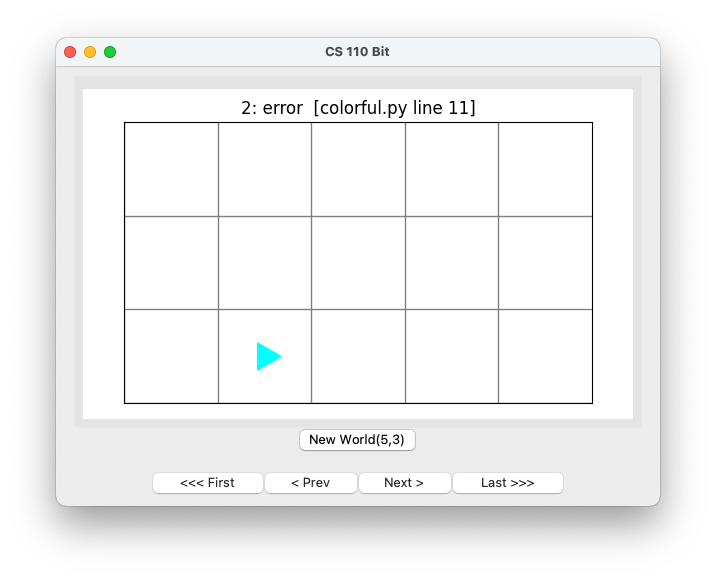The error message at the top of the window doesn’t tell you very much. But if you look at the bottom of PyCharm you will see this: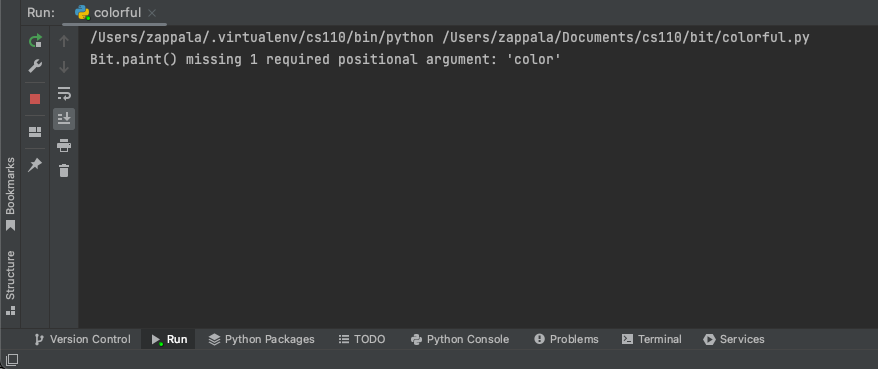When you see this message:

``Bit.paint() missing 1 required positional argument: 'color'``

This means that you tried to call `bit.paint()` but you are missing the required argument — a color you want to paint. You need to use `'green'`, `'red'`, or `'blue'` as the color.

## Solving a problem

Download grassy_field.zip and put its contents into your `bit` folder. You should have a folder called `grassy_field` with a file called `grassy_field.py` and a folder called `worlds`: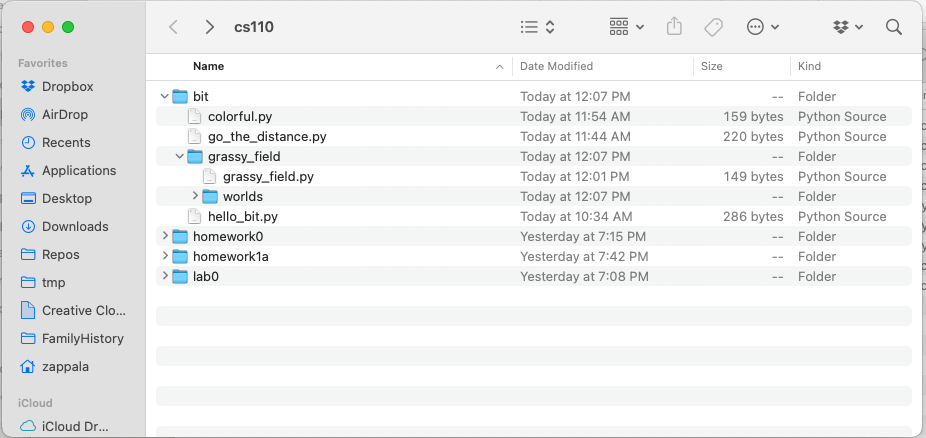Inside of `grassy_field.py` you will see this code:

``````from byubit import Bit

@Bit.worlds("grassy_field")
def make_sky(bit):
bit.paint('blue')

if __name__ == '__main__':
make_sky(Bit.new_bit)``````

In this example, Bit starts in a world called `grassy_field`. The files needed for this world are located in the `worlds` folder.

The starting world for Bit looks like this: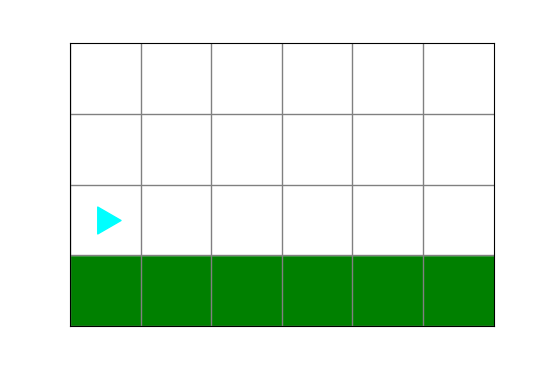The ending world should look like this:However, when you run this code, you will see a comparison error: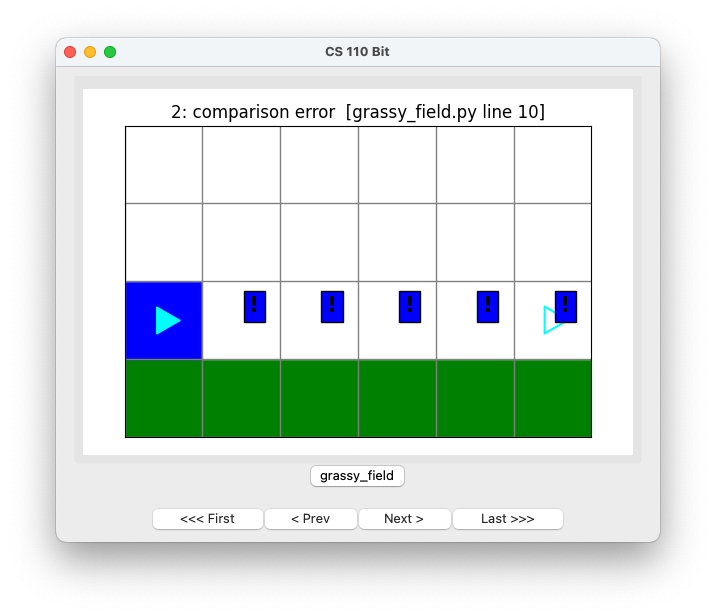What you see here is:

• `exclamation mark with a blue background` — this means that square should be blue
• `empty Bit at the far right` — this is where Bit should be located and what direction Bit should be pointing

Can you fix the code so that it works properly?Hopefully you realized you need to call the `bit.move()` and `bit.paint()` functions:

``````def make_sky(bit):
bit.paint('blue')
bit.move()
bit.paint('blue')
bit.move()
bit.paint('blue')
bit.move()
bit.paint('blue')
bit.move()
bit.paint('blue')
bit.move()
bit.paint('blue')``````